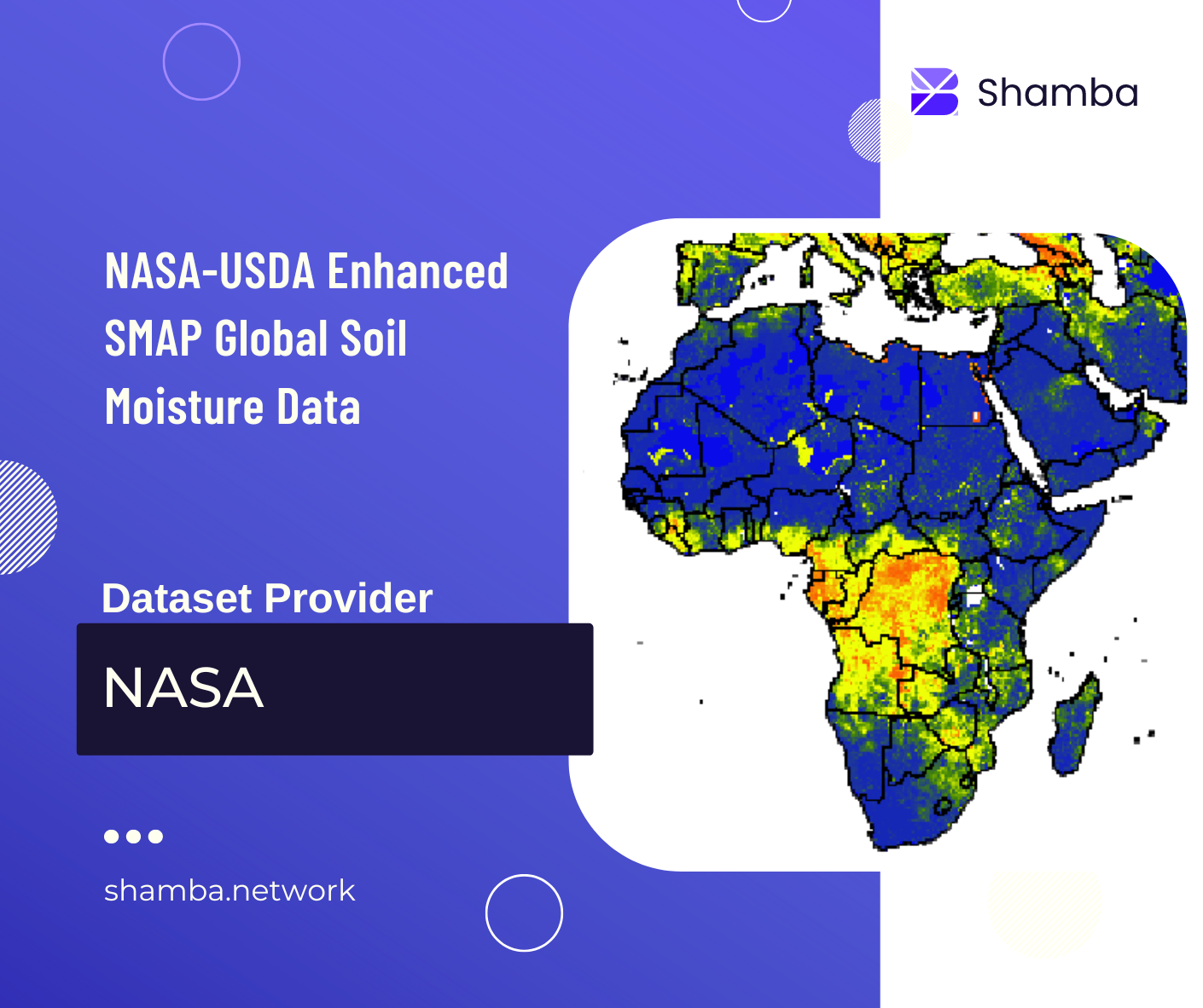# Data Model

The Shamba oracle currently supports running two types of analytics on geospatial data. Below we describe each of these including their inputs and output.

## Descriptive Statistics Analysis

This analysis aims at getting descriptive statistics. Given an area of interest and a dataset to reference, this analysis returns standard metrics such as maximum, minimum, mean, median and variance. These descriptive statistics are useful for setting thresholds and triggers in many parametric smart contracts. The required statistical metric is specified in the “agg_x” parameter.

Inputs:

• “agg_x”: “string”,

• “dataset_code”: “string”,

• “selected_band”: “string”,

• “geometry”: {json},

• “start_date”: “string”,

• “end_date”: “string”,

• “image_scale”: integer,

Outputs:

• int256

## Fire Analysis

This analysis aims at determining if an area of interest falls within a detected fire. It performs a topology analysis to determine if a fire extends to a specified area of interest. Given an area of interest and a dataset to reference, this analysis returns a boolean indicating whether a fire was detected or not. Boolean values (1 - TRUE, 9 - FALSE).

Inputs:

• “dataset_code”: “string”,

• “selected_band”: “string”,

• “geometry”: {json},

• “start_date”: “string”,

• “end_date”: “string”,

• “image_scale”: integer,

Outputs:

• uint256 (1 - TRUE, 9 - FALSE)

## Thematic Areas & Dataset

The Shamba oracle uses regularly updated geospatial data from various global providers. Different providers use different algorithms to compute the same metric, so it is recommended that users go through the literature for each dataset. Normalization has been performed where necessary to standardize values from different datasets under a common theme.

### Soil MoistureProperties Value Pixel Size (metres) 10000 Image Capture Frequency Monthly Dataset Code SMAP_SM Bands ssm Units mm Geographic Coverage Global Read More Link_8